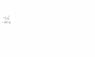# Optimization of 2 eqns

• strokebow
In summary, the goal of the conversation was to optimize two equations (S and x), with constants h and t and variables w and j. The equations were provided and the individual had attempted to rearrange them to make j the subject, but was unable to do so. They were looking for help in finding the minimum/maximum values for S and x by plotting them over a range of h and w. It was suggested to try fixing j as a positive or negative number and finding the corresponding values for w that would minimize x or maximize S.

## Homework Statement

I need to optimise a couple of equations.
I want maximum S for minimum x.

Constants:
h, t

Variables:
w, j

## Homework Equations

S = ( (j) / (j + 0.5*w) )^2 [Eqn 1]
x = (const) * (j / w) [Eqn 2]
[See attachment]

## The Attempt at a Solution

Well...
I've tried to re-arrange [Eqn 1] to make j the subject but I cannot. I get:
((j)^2) * ( 1 - S + ((S*w)/j) ) = (S*(w^2))/4

I thought that if I rearrange both equations for j or w and set them equal to each other then I can try to find the min/max of the equation (for the other variable).

thanks

**Edit Oops... posted in the wrong forum sub category. This is not homework nor coursework. This is a personal project. Either way, I could do with some help :-)

#### Attachments

•temp.jpg
2.4 KB · Views: 394
Last edited:
I'm thinking... is it even possible?

Surely, I can plot S and x over a range of h and w and find where S is maximum and r is minimum??

Might be instructive to go through some mental gyrations. For example, assume j is a fixed positive number and figure out what value of w will minimize x or maximize S. Then assume j is a fixed negative number and figure out what value of w will minimize x or maximize S. You should be able to zero in rather quickly on what you are looking for.